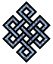#fail2ban bad ip database: ip 85.152.27.19

### | ip database | live view | stats | report | help | api key:

 ip: 85.152.27.19 hostname: cm-staticIP-85-152-27-19.telecable.es country:[ES] Spain first reported: 20.11.2018 19:17.57 GMT+0200 last reported: 23.04.2019 11:24.12 GMT+0200 time period: 153d 15h 06m 15s total reports: 12 reported by: 3 host(s) filter(s): ssh (9) ssh (3) tor exit node no badips.com db Lookup## port scan of '85.152.27.19':

[-hide]
```# Nmap 6.40 scan initiated Tue Nov 20 19:18:01 2018 as: /usr/bin/nmap -sU -sS -O 85.152.27.19
Nmap scan report for cm-staticIP-85-152-27-19.telecable.es (85.152.27.19)
Host is up (0.051s latency).
Not shown: 1000 open|filtered ports, 992 filtered ports
PORT      STATE  SERVICE
22/tcp    open   ssh
80/tcp    open   http
81/tcp    closed hosts2-ns
113/tcp   closed ident
443/tcp   open   https
1723/tcp  open   pptp
8008/tcp  open   http
60443/tcp open   unknown
No exact OS matches for host (If you know what OS is running on it, see http://nmap.org/submit/ ).
TCP/IP fingerprint:
OS:SCAN(V=6.40%E=4%D=11/20%OT=22%CT=81%CU=%PV=N%G=Y%TM=5BF45002%P=x86_64-pc
OS:-linux-gnu)SEQ(SP=105%GCD=3%ISR=107%TI=Z%TS=8)OPS(O1=M5B4ST11NW7%O2=M5B4
OS:ST11NW7%O3=M5B4NNT11NW7%O4=M5B4ST11NW7%O5=M5B4ST11NW7%O6=M5B4ST11)WIN(W1
OS:=7120%W2=7120%W3=7120%W4=7120%W5=7120%W6=7120)ECN(R=Y%DF=Y%TG=40%W=7210%
OS:O=M5B4NNSNW7%CC=Y%Q=)ECN(R=N)T1(R=Y%DF=Y%TG=40%S=O%A=S+%F=AS%RD=0%Q=)T1(
OS:R=N)T2(R=N)T3(R=N)T4(R=N)T5(R=Y%DF=Y%TG=40%W=0%S=Z%A=S+%F=AR%O=%RD=0%Q=)
OS:T5(R=N)T6(R=N)T7(R=N)U1(R=N)IE(R=N)

OS detection performed. Please report any incorrect results at http://nmap.org/submit/ .
# Nmap done at Tue Nov 20 19:18:42 2018 -- 1 IP address (1 host up) scanned in 40.71 seconds
```
```Σ = 94 | Δt = 0.0074129104614258s
```